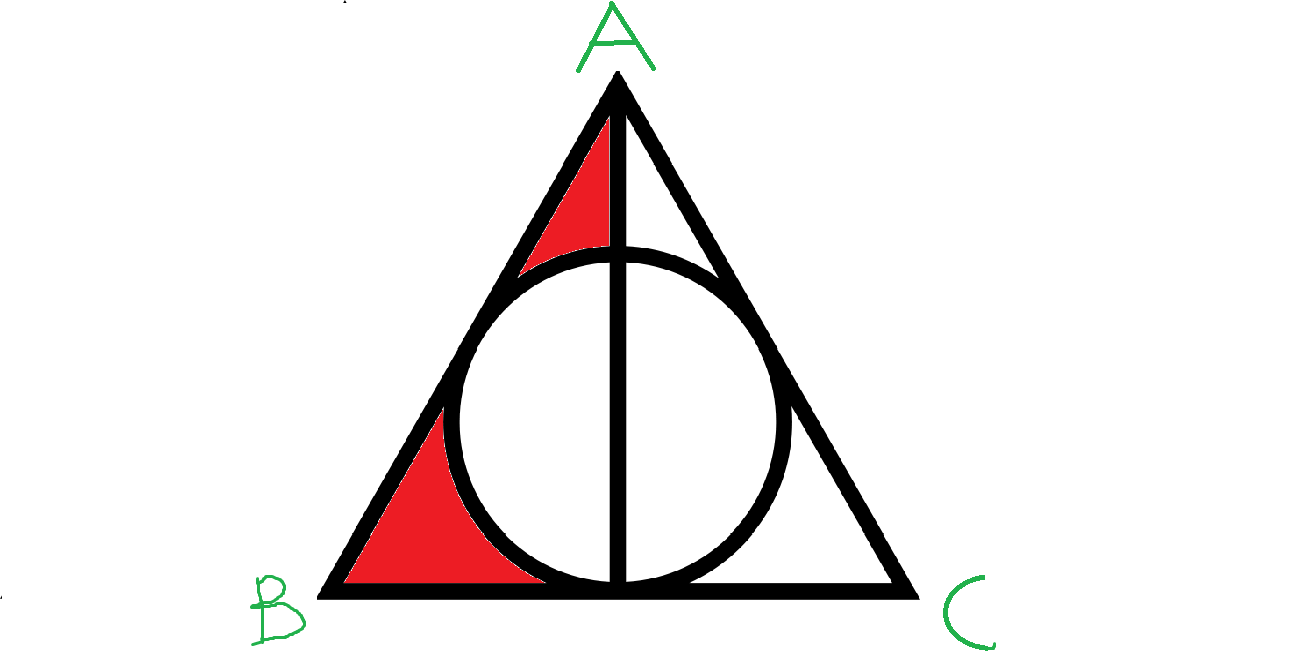# Harry Potter's Magical Geometry!In the given figure , $\Delta ABC$ is an equilateral triangle with side as 1 unit.

The given circle is the in-circle of $\Delta ABC$

If Area of red colored region can be expressed as:

$\dfrac{a\sqrt{b} - c}{d}$ where $a,b,c,d\in \mathbb{N}$ ,$b$ is independent of a perfect square ,

Find $a + b + c + d$

Details and Assumptions:-

• Take $\pi$ as $\dfrac{22}{7}$.
×